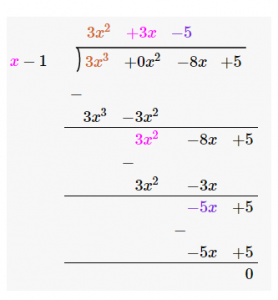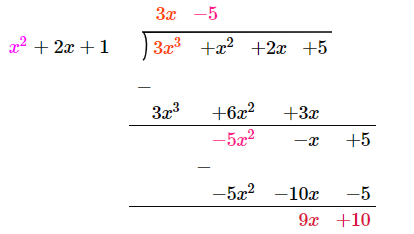Courses

# Division Algorithm for Polynomials Notes | EduRev

## Class 10 : Division Algorithm for Polynomials Notes | EduRev

The document Division Algorithm for Polynomials Notes | EduRev is a part of the Class 10 Course Mathematics (Maths) Class 10.
All you need of Class 10 at this link: Class 10

The polynomial division involves the division of one polynomial by another. The division of polynomials can be between two monomials, a polynomial and a monomial or between two polynomials. Before learning how to divide polynomials, let’s have a brief introduction to the definition of polynomial and its related terms.

Polynomial

A polynomial is an algebraic expression of the type anx+ an−1xn−1+…………………a2x+ a1x + a0, where “n” is either 0 or positive variables and real coefficients.
In this expression, an, an−1…..a1,a0 are coefficients of the terms of the polynomial.
The highest power of x in the above expression, i.e. n is known as the degree of the polynomial.
If p(x) represents a polynomial and x = k such that p(k) = 0 then k is the root of the given polynomial.

Example: Given a polynomial equation, p(x)=x2–x–2. Find the zeros of the equation.
Solution:
Given Polynomial, p(x)=x2–x–2
Zeros of the equation is given by:
x2–2x+x–2=0
x(x−2)+1(x–2)
(x+1)(x−2)=0
⇒ x=−1
Or, x=2
Thus, -1 and 2 are zeros of the given polynomial.

It is to be noted that the highest power(degree) of the polynomial gives the maximum number of zeros of the polynomial.

Division of Polynomial

The division is the process of splitting a quantity into equal amounts. In terms of mathematics, the process of repeated subtraction or the reverse operation of multiplication is termed as division. For example, when 20 is divided by 4 we get 5 as the result since 4 is subtracted 5 times from 20. The four basic operations viz. addition, subtraction, multiplication and division can also be performed on algebraic expressions. Let us understand the process and different methods of dividing polynomials and algebraic expressions.

Types of Polynomial Division

For dividing polynomials, generally, three cases can arise:

• Division of a monomial by another monomial
• Division of a polynomial by monomial
• Division of a polynomial by binomial
• Division of a polynomial by another polynomial

Let us discuss all these cases one by one:

Division of a monomial by another monomial

Consider the algebraic expression 40x2 is to be divided by 10x then
40x2/10x = (2×2×5×2×x×x)/(2×5×x)
Since 2, 5 and x are common in both the numerator and the denominator.
Hence, 40x2/10x = 4x

Division of a polynomial by monomial

The second case is when a polynomial is to be divided by a monomial. For dividing polynomials, each term of the polynomial is separately divided by the monomial (as described above) and the quotient of each division is added to get the result. Consider the following example:

Example: Divide 24x3 – 12xy + 9x by 3x.
Solution: The given expression 24x3 – 12xy + 9x has three terms viz. 24x3, – 12xy and 9x. F
or dividing the polynomial with a monomial, each term is separately divided as shown below:
(24x3–12xy+9x)/3x = (24x3/3x)–(12xy/3x)+(9x/3x) = 8x2–4y+3

Division of a Polynomial by Binomial

As we know, binomial is an expression with two terms. Dividing a polynomial by binomial can be done easily. Here, first we need to write the given polynomial in standard form. Now, using the long division method, we can divide the polynomial as given below.

Example: Divide 3x3 – 8x + 5 by x – 1.
Solution: The Dividend is 3x3 – 8x + 5 and the divisor is x – 1.
After this, the leading term of the dividend is divided by the leading term of the divisor i.e. 3x3 ÷ x =3x2.
This result is multiplied by the divisor i.e. 3x2(x -1) = 3x3 -3x2 and it is subtracted from the divisor.
Now again, this result is treated as a dividend and the same steps are repeated until the remainder becomes zero or its degree becomes less than that of the divisor as shown below.Division of Polynomial by Another Polynomial

For dividing a polynomial with another polynomial, the polynomial is written in standard form i.e. the terms of the dividend and the divisor are arranged in decreasing order of their degrees. The method to solve these types of divisions is “Long division”. In algebra, an algorithm for dividing a polynomial by another polynomial of the same or lower degree is called polynomial long division. It is the generalised version of the familiar arithmetic technique called long division. Let us take an example.
Example: Divide  x2 + 2x + 3x3 + 5 by 1 + 2x + x2.
Solution:
Let us arrange the polynomial to be divided in the standard form.
3x3 + x2 + 2x + 5
Divisor = x2 + 2x + 1
Using the method of long division of polynomials, let us divide 3x3 + x2 + 2x + 5 by x2 + 2x + 1.Polynomial Division Algorithm

If p(x) and g(x) are any two polynomials with g(x) ≠ 0, then we can find polynomials q(x) and r(x) such that
p(x) = g(x) × q(x) + r(x)
Here,
r(x) = 0 or degree of r(x) < degree of g(x)
This result is called the Division Algorithm for polynomials.
From the previous example, we can verify the polynomial division algorithm as:
p(x) = 3x3 + x2 + 2x + 5
g(x) = x2 + 2x + 1
Also, quotient = q(x) = 3x – 1
remainder = r(x) = 9x + 10

Now,
g(x) × q(x) + r(x) = (x2 + 2x + 1) × (3x – 5) + (9x + 10)
= 3x3 + 6x2 + 3x – 5x2 – 10x – 5 + 9x + 10
= 3x3 + x2 + 2x + 5
= p(x)
Hence, the division algorithm is verified.

Polynomial Division Questions

1. If the polynomial x4 – 6x3 + 16x2 – 25x + 10 is divided by another polynomial x2 – 2x + k, the remainder comes out to be x + a, find k and a.
2. Divide the polynomial 2t4 + 3t3 – 2t2 – 9t – 12 by t2 – 3.

Find all the zeroes of 2x4 – 3x3 – 3x2 + 6x – 2, if two of its zeroes are √2 and −√2.

Offer running on EduRev: Apply code STAYHOME200 to get INR 200 off on our premium plan EduRev Infinity!

## Mathematics (Maths) Class 10

51 videos|346 docs|103 tests

,

,

,

,

,

,

,

,

,

,

,

,

,

,

,

,

,

,

,

,

,

;